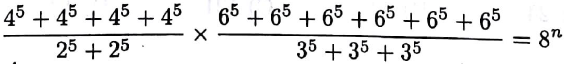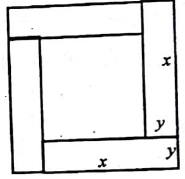Tuesday, November 24, 2020
Home > NMTC > Sample Paper For NMTC Stage 1 Sub Junior Level | Class 7 and 8

# Sample Paper For NMTC Stage 1 Sub Junior Level | Class 7 and 8NMTC Stage 1 Sample Questions
Primary LevelSub Junior LevelJunior Level

Hi students, welcome to Amans Maths Blogs (AMB). On this post you will get Sample Paper For NMTC Stage 1 Sub Junior Level (Class 7 and 8). The questions of this sample paper are published on this post, it will help you to understand the level of questions asked in NMTC Stage 1 in Sub Junior Level. So lets start with the questions as below:

Sample Paper For NMTC Stage 1 Sub Junior Level Question No 1

In a queue, Amar is 10th from the front while Akbar is 25th from behind and Antony is just in the middle of the two. If there be 50 persons in the queue, then the position occupied by Antony from the front is

Options

A. 16th

B. 18th

C. 19th

D. 30th

Sample Paper For NMTC Stage 1 Class 7 and 8 Question No 2

How many times should 2002 be subtracted from 112113 to get remainder 2003?

Options

A. 53

B. 55

C. 65

D. 68

Sample Paper For NMTC Stage 1 Class 7 and 8 Question No 3

A students starts at the year 2001 and counts backwards, 8 at a time, giving the sequence of years 2001, 1993, 1985, …. A year which she will count is

Options

A. 1841

B. 1901

C. 1923

D. 1903

Sample Paper For NMTC Stage 1 Class 7 and 8 Question No 4

In the sequence, 1, 22, 333, 4444,…, the nth term has n number of n’s. Then, the sum of the number of digits in the 100th term is

Options

A. 100

B. 300

C. 10000

D. 100100

Sample Paper For NMTC Stage 1 Sub Junior Level Question No 5

Scientist A has invented a device for cars to save petrol by 20%. Scientist B’s invention saves 30%, invention by C saves 40%. The three inventions are independent in effect. If all the three devices are used, how much petrol can one save?

Options

A. 90%

B. 140%

C. 66.4%

D. 36.6%

Sample Paper For NMTC Stage 1 Class 7 and 8 Question No 6

A watch is set right at 3 pm. It looses 20 minutes in 24 hours. The true time when the watch shows 2 pm on fourth day is

Options

A. 3 pm

B. 4 : 19 pm

C. 3 : 19 pm

D. 4 pm

Sample Paper For NMTC Stage 1 Class 7 and 8 Question No 7

The value of n in the equation below isOptions

A. 1

B. 2

C. 3

D. 4

Sample Paper For NMTC Stage 1 Class 7 and 8 Question No 8

The total numbers of powers of prime factors in the expression 67 x 3517 x 3327 is

Options

A. 24

B. 44

C. 121

D. 102

Sample Paper For NMTC Stage 1 Class 7 and 8 Question No 9

If a – 1 = b + 2 = c – 3 = d + 4, then the largest among a, b, c, d is

Options

A. a

B. b

C. c

D. d

Sample Paper For NMTC Stage 1 Sub Junior Level Question No 10

Given single digits, A, B, C, D, E, X and Y such that A + B + C + D + E = XY where the two-digit number XY has a value of 10X + Y. To make XY a greatest value, the value of Y should be

Options

A. 4

B. 3

C. 2

D. 1

Sample Paper For NMTC Stage 1 Class 7 and 8 Question No 11

In a triangle ABC, BD bisects angle B. If angle A = (2/3) of angle B and angle B = 3 times of angle C, then the angle BDC is

Options

A. 75 degree

B. 105 degree

C. 90 degree

D. 120 degree

Sample Paper For NMTC Stage 1 Class 7 and 8 Question No 12

The area of the largest possible square inscribed in a circle of unit radius is

Options

A. 2

B. 2Pi

C. 4

D. 2√2Pi

Sample Paper For NMTC Stage 1 Class 7 and 8 Question No 13

The small square is surrounded by four congruent rectangles as shown to form a large square. If the perimeter of each rectangle is 16, the area of the large square isOptions

A. 64

B. 256

C. 32

D. 224

Sample Paper For NMTC Stage 1 Sub Junior Level Question No 14

Which one of the following is true?

(i) If A is a brother of B, then B is a brother of A

(ii) If A likes B and B likes C, then A likes C

(iii) If A ≠ B and B ≠ C, then A ≠ C.

Options

A. Both (i) and (ii)

B. (ii) only

C. All the three

D. None of them

Sample Paper For NMTC Stage 1 Class 7 and 8 Question No 15

Five year ago, the average age of A, B, C and D was 45 years. With E joining them now, the average age of all the five is 49 years. The present age of E is

Options

A. 40

B. 45

C. 42

D. 48

Sample Paper For NMTC Stage 1 Class 7 and 8 Question No 16

The median of the numbers -4, 2, 0, -6, 5, 8 is

Options

A. 1

B. 2

C. 0

D. -4

Sample Paper For NMTC Stage 1 Sub Junior Level Question No 17

Find the smallest number which when divided by 10 leaves a remainder of 9, when divided by 9 leaves a remainder of 8, when divided by 8 leaves a remainder of 7, …etc down to where when divided by 2 it leaves a remainder 1.

Options

A. 2356

B. 2519

C. 2825

D. 2864

Sample Paper For NMTC Stage 1 Class 7 and 8 Question No 18

The table below defines an operation (*). For example, from the table, we find a x c = d and b x d = b. If b x x = a, then the value of x x isOptions

A. a

B. b

C. c

D. d

Sample Paper For NMTC Stage 1 Class 7 and 8 Question No 19

A cube of side 6 cm is cut into a number of cubes, each of side 2 cm. The number of cubes will be

Options

A. 27

B. 9

C. 6

D. 3

Sample Paper For NMTC Stage 1 Sub Junior Level Question No 20

D, A, O, B and C are points on a line. Circles are drawn with O, A and B as centers as shown below. If the radius of smallest circle is 1 cm, then the length of CD isOptions

A. 3 cm

B. 4 cm

C. 6 cm

D. 6 cm

Sample Paper For NMTC Stage 1 Class 7 and 8 Question No 21

If a + b + c = 0, then find the value of (a2 + b2 + c2)/(a2b2 + b2c2 + c2a2) is

Options

A. 2

B. 1

C. 3

D. 4

Sample Paper For NMTC Stage 1 Class 7 and 8 Question No 22

A question paper has n questions (n > 20). Out of the first 20, a students answers 15 correctly and out of the remaining he answers one-third correctly. If all questions carry equal marks, and the student’s total scores is 50%, then the total number of questions is

Options

A. 35

B. 65

C. 50

D. 40

Sample Paper For NMTC Stage 1 Sub Junior Level Question No 23

Instead of multiplying a given number by 8/19, a students divide it by 8/19. His answer was 297 more than the correct answer. The given number was

Options

A. 8

B. 19

C. 152

D. 64

1. B

2. B

3. A

4. A

5. C

6. C

7. D

8. D

9. C

10. C

11. B

12. B

13. A

14. D

15. B

16. A

17. B

18. B

19. A

20. A

21. D

22. C

23. C

NMTC Stage 1 Sample Questions